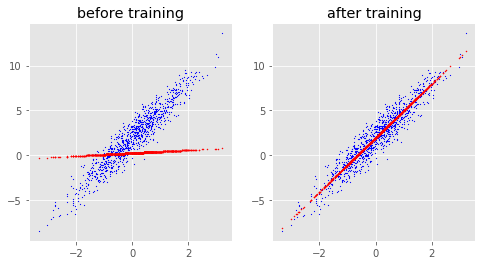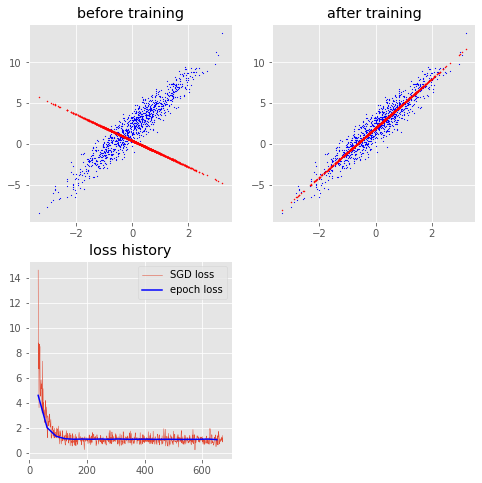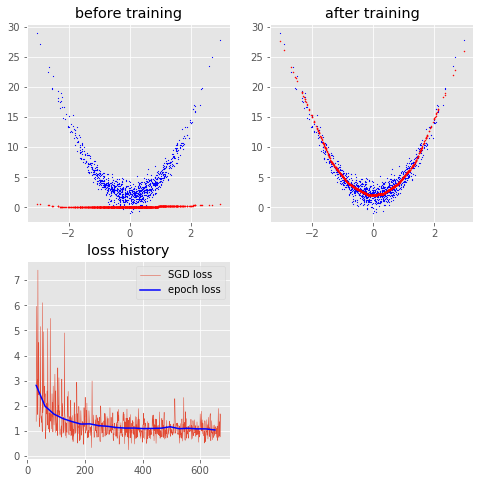# Playing with TensorFlow 2.0¶

July 29, 2019

I had some free time after work, so I figured I would write something about TensorFlow 2.0, the newest version of the most popular deep learning library in existence today. The new TensorFlow is interesting because it has eager execusion by default. This enables effortless conversion between the tf.tensor the numpy array, making tensor value checks possible at any moment during development. How amazing is that! Imagine the improved productivity this can bring. To install the beta version, let's creating a virtual environment (highly recommended), in which we run:

pip install tensorflow==2.0.0-beta1

Let's import some libraries as usual.

In :
from __future__ import absolute_import, division, print_function, unicode_literals
import tensorflow as tf
from tensorflow.keras import layers
import matplotlib.pyplot as plt
plt.style.use('ggplot')
import numpy as np
import math
print(tf.__version__)

2.0.0-beta1


A nice thing about TensorFlow 2.0 is that a lot of old messy libaries are cleaned, making it more numpy-like. Numpy has always been my favorite Python library, and now TensorFlow is not far behind. Sure enough, just like np.random.normal, we can use tf.random.normal to create a random tensor. Let's generate some data first.

In :
# generate linear data (X,y)
true_W = 3.0
true_b = 2.0
n = 1000
X  = tf.random.normal(shape=(n,1))
noise   = tf.random.normal(shape=(n,1))
y = X * true_W + true_b + noise
print('Data has shape:', X.shape)
print('Label has shape:', y.shape)

Data has shape: (1000, 1)
Label has shape: (1000, 1)


## Method 1 - Using compile and fit¶

The reason I love TensorFlow (and Python in general) is its object-oriented expressiveness. To create a custom layer, we can simply build on top of the pre-built keras classes like Layer and Model. Class inheritance enables the use of the compile and fit method directly without expicitly writing them. Mini-batch gradient descent is a free bonus.

In :
class LinearLayer(layers.Layer):
def __init__(self, units=1):
super(LinearLayer, self).__init__()
self.units = units

def build(self, input_shape):
shape=(int(input_shape[-1]),
self.units))
shape=(1,self.units))

def call(self, input_tensor):
return tf.matmul(input_tensor, self.kernel) + self.bias

In :
class LinearModel(tf.keras.Model):
def __init__(self):
super(LinearModel, self).__init__()
self.mylayer = LinearLayer()

def call(self, x):
x = self.mylayer(x)
return x

In :
# adding subplot to matplotlib figure
def add_scatter(fig, model, X, y, title='', axis=[1,2,1]):
yhat = model(X).numpy()
X, y = X.numpy(), y.numpy()
ax.scatter(X, y, c='b', s=0.5)
ax.scatter(X, yhat, c='r', s=1)
ax.set_title(title)

In :
fig = plt.figure(figsize=(8,4))
model = LinearModel()
optimizer = tf.keras.optimizers.SGD(learning_rate=0.01)

model.compile(
optimizer = optimizer,
loss = tf.losses.mean_squared_error,
metrics = ['mse']
)

add_scatter(fig, model, X, y, title='before training', axis=(1,2,1))

model.fit(
x=X,
y=y,
epochs=100,
verbose=0
)

add_scatter(fig, model, X, y, title='after training', axis=(1,2,2))## Method 2 - More Customization¶

The incredible flexibility of TensorFlow enables more customized models to be built. We can be as customized as we want. Instead of using the fit function, we can write them explicitly. First we start by implementing the mini-batch gradient descent.

In :
def random_mini_batches(X, y, mini_batch_size=32, seed=0):
n = X.shape
mini_batches = []

# shuffle data
df = tf.concat(values=[X,y], axis=1)
shuffled_df = tf.random.shuffle(df, seed=seed) # love the similarity to numpy
shuffled_X = tf.reshape(shuffled_df[:,0], shape=(n,1))
shuffled_y = tf.reshape(shuffled_df[:,1], shape=(n,1))

# randomly subsetting into minibatches (not including the end case)
num_complete_minibatches = math.floor(n/mini_batch_size)
for k in range(num_complete_minibatches):
mini_batch_X = shuffled_X[k*mini_batch_size : k*mini_batch_size + mini_batch_size,:]
mini_batch_y = shuffled_y[k*mini_batch_size : k*mini_batch_size + mini_batch_size,:]
mini_batch = (mini_batch_X, mini_batch_y)
mini_batches.append(mini_batch)

# last minibatch
if n % mini_batch_size != 0:
mini_batch_X = shuffled_X[num_complete_minibatches*mini_batch_size : n,:]
mini_batch_y = shuffled_y[num_complete_minibatches*mini_batch_size : n,:]
mini_batch = (mini_batch_X, mini_batch_y)
mini_batches.append(mini_batch)

return mini_batches

In :
# specify loss function
def loss(y_hat, y):
residual = y_hat - y
return tf.reduce_mean(tf.square(residual))

In :
# define the gradient function
loss_value = loss(model(X), y)

In :
def train(model, X, y, num_epochs=10, mini_batch_size=128, learning_rate=0.01, verbose=0):
costs = []
mini_batch_costs = []
n = X.shape
num_mini_batches = int(n/mini_batch_size)
optimizer = tf.keras.optimizers.SGD(learning_rate=learning_rate)
fig = plt.figure(figsize=(8,8))
add_scatter(fig, model, X, y, title='before training', axis=(2,2,1))

for epoch in range(num_epochs):
mini_batch_cost = 0
batch = random_mini_batches(X, y, mini_batch_size)

for mini_batch in batch:
(mini_batch_X, mini_batch_y) = mini_batch
mini_batch_costs.append(temp_cost.numpy())
mini_batch_cost += temp_cost / num_mini_batches

costs.append(mini_batch_cost.numpy()) # append final cost

if verbose == 1 and epoch % 10 == 0:
print("Epoch %i - Cost: %1.2f" % (epoch, mini_batch_cost))
print("--------------------------------------")

costs.append(loss(model(X),y).numpy()) # append final cost
# plot loss history
add_scatter(fig, model, X, y, title='after training', axis=(2,2,2))
m = len(mini_batch_costs)
ax.plot(range(num_mini_batches, m), mini_batch_costs[num_mini_batches:], linewidth='0.5')
ax.plot([x*num_mini_batches for x in range(1,num_epochs+1)], costs[1:], c='b')
ax.set_title('loss history')
ax.legend(['SGD loss', 'epoch loss']) # stochastic gradient descent (SGD)
plt.show()

In :
model = LinearModel()
train(model, X, y, mini_batch_size=32, num_epochs=21, learning_rate=0.01, verbose=1)

Epoch 0 - Cost: 15.76
--------------------------------------
Epoch 10 - Cost: 1.11
--------------------------------------
Epoch 20 - Cost: 1.10
--------------------------------------Why do I start with linear regression? Because it can be easily generalize to a neural network or arbitrary complexity! Enjoy.

In :
class NonLinearModel(tf.keras.Model):
def __init__(self):
super(NonLinearModel, self).__init__()
self.mylayer1 = LinearLayer(10)
self.mylayer2 = LinearLayer(5)
self.mylayer3 = LinearLayer(1)

def call(self, x):
x = self.mylayer1(x)
x = tf.nn.relu(x)
x = self.mylayer2(x)
x = tf.nn.relu(x)
x = self.mylayer3(x)
return tf.nn.relu(x)

In :
# generate quadratic data (X,y)
true_W = 3.0
true_b = 2.0
n = 1000
X  = tf.random.normal(shape=(n,1))
noise   = tf.random.normal(shape=(n,1))
y = X**2 * true_W + true_b + noise

In :
model = NonLinearModel()
train(model, X, y, mini_batch_size=32, num_epochs=21, learning_rate=0.01, verbose=1)

Epoch 0 - Cost: 11.50
--------------------------------------
Epoch 10 - Cost: 1.13
--------------------------------------
Epoch 20 - Cost: 1.08
--------------------------------------